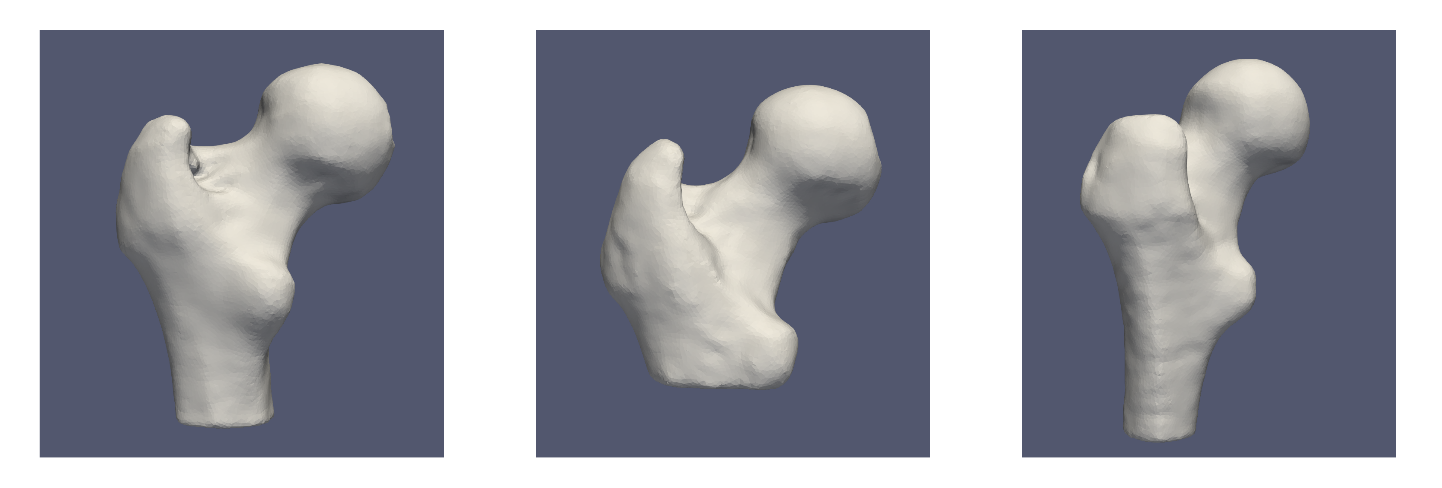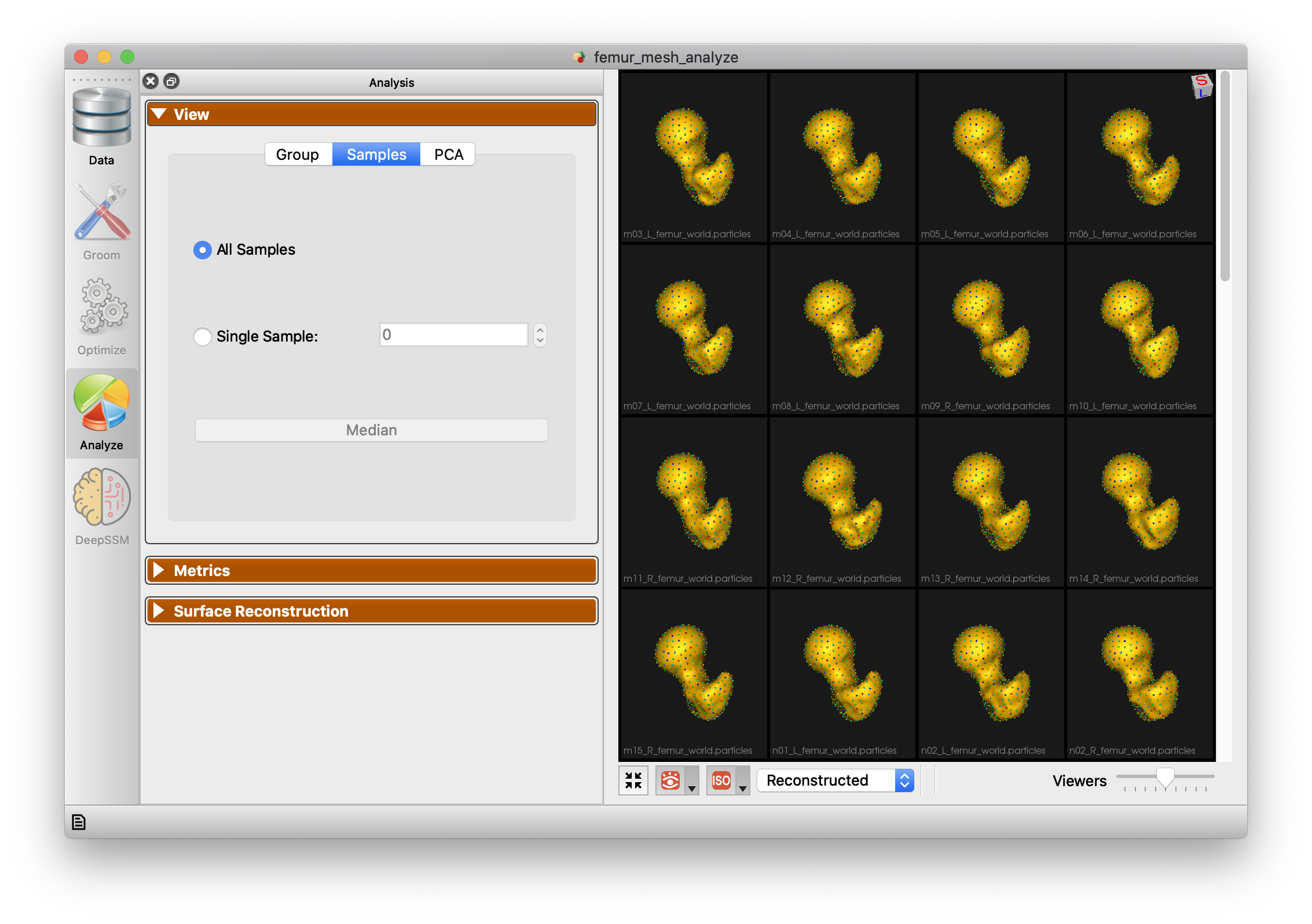# Femur-Mesh: Shape Model directly from Mesh

## What and Where is the Use Case?

This use case demonstrates using ShapeWorks tools to perform the following.

• Build a shape model where shapes are given as triangular surface meshes
• Groom a dataset from meshes, and obtain a SSM without converting the meshes to a different representation. This requires significantly lower RAM.

The use case is located at: Examples/Python/femur_mesh.py## Running the Use Case

To run the use case, run RunUseCase.py (in Examples/Python/).

$cd /path/to/shapeworks/Examples/Python$ python RunUseCase.py --use_case femur_mesh


This calls femur_mesh.py (in Examples/Python/) to perform the following.

• Optimizes particle distribution (i.e., the shape/correspondence model) by calling optimization functions in OptimizeUtils.py (in Examples/Python/). See Optimizing Shape Model for details about algorithmic parameters for optimizing the shape model.
• Launches ShapeWorksStudio to visualize the use case results (i.e., the optimized shape model and the groomed data) by calling functions in AnalyzeUtils.py (in Examples/Python/).

## Grooming Data

In this use case, we download pre-groomed data. The grooming process used for this data can be explored in Libs/Mesh/GroomFemur.cpp.

1. Clip: Because the femur meshes vary in shaft lengths, we clip the lengths of all the femurs to that of the shortest one.
2. Reflect Meshes: In this use case, we often have both right and left femur surface meshes. To align all the femurs, we choose one side to reflect both the image and mesh.
3. Scale: ShapeWorks is not size invariant and requires a sufficient scale.
4. Centering: This factors out translations to reduce the risk of misalignment.
5. Alignment + Cropping: This process is to further ensure that all the shafts are cropped at the same cutting plane.

## Optimizing Shape Model

Below are the default optimization parameters when running this use case. For a description of the optimize tool and its algorithmic parameters, see: How to Optimize Your Shape Model.

\$ python RunUseCase.py --use_case femur_mesh

{
"number_of_particles": 1024,
"use_shape_statistics_after": 0,
"use_normals": 0,
"normal_weight": 0.0,
"checkpointing_interval" : 10000,
"keep_checkpoints" : 0,
"iterations_per_split" : 4000,
"optimization_iterations" : 500,
"starting_regularization" : 10,
"ending_regularization" : 1,
"recompute_regularization_interval" : 1,
"domains_per_shape" : 1,
"domain_type" : 'mesh',
"relative_weighting" : 10,
"initial_relative_weighting" : 1,
"procrustes_interval" : 1,
"procrustes_scaling" : 1,
"save_init_splits" : 0,
"verbosity" : 2,
}


## Analyzing Shape Model

ShapeWorksStudio visualizes/analyzes the optimized particle-based shape model by visualizing the mean shape, individual shape samples, and the shape modes of variations. For more information, see: How to Analyze Your Shape Model?.

Here is the mean shape of the optimized shape mode using single-scale optimization.Here are femur samples with their optimized correspondences.Here is a video showing the shape modes of variation (computed using principal component analysis - PCA) of the femur dataset using single-scale optimization.